# Bayesian inference: are parameters fixed or random?

Yesterday I had an interesting discussion with a friend about how parameters are thought of in Bayesian inference. Coming from a predominantly frequentist statistical education, I had somewhere along the line picked up the notion that for Bayesians, like frequentists, the model parameters (their true values) are unknown but fixed quantities. The prior distribution then represents the prior belief about the location of this fixed value, before the data are seen. Thus the prior distribution represents our uncertainty about the location of the unknown, but fixed, parameter value.

Another view however is that the parameter value used to generate the data that are obtained in your study is just one drawn parameter value, where the draw is from some distribution (the prior). Here, as far as I understand, the prior is being used to represent the idea that across some universe of potential studies, each with data generated conditional on the parameter value sampled (by nature) from the prior in that study. For example, in the clinical world where we are interested in the effects of treatments or interventions, we often try and estimate the effect using a randomized trial. If one were to repeat the study again, later in time, the (true) treatment effect being estimated would not be the same as in the first. This change in true treatment effect could be due to various changes beyond the control of the investigator, such as temporal changes in characteristics of the population which somehow cause the (true) treatment effect to change. In this view of the world, the prior distribution represents (perhaps entirely, or partially) the distribution of these different true treatment effects.

To me, if one believes that the treatment effect is varying as certain inputs change (e.g. temporal changes), and one wants to explicitly model such variation, then this requires a modification of the model to a hierarchical model. Here we would assume that each study’s data is generated conditional on its own parameter value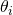. We would then specify a distribution for the, for example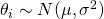. We would then need to specify a prior for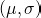, with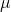representing the overall mean true treatment effect across this universe of (potential) studies, while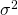represents the variability of true treatment effects across the universe of studies. In such a model, I would not personally call theas parameters, but rather as random or latent effects.

Part of the confusion/apparent disagreement here could simply be due to terminology. In ’Bayesian Data Analysis’, when describing hierarchical models, Gelman et al refer to thein a hierarchical model as parameters, and then refer to the distributional assumption made about them (e.g.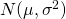) as being a ‘prior (or population) distribution’. The prior for what I have in the above defined as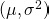is then labelled a ‘hyperprior distribution’. At least to my mind, this is a confusing terminology. The distributional assumption for the(which Gelman et al refer to as parameters, but I would refer to as random-effects) is a structural assumption of the model. The prior distribution for the parameters(which I would call a prior, but Gelman et al refer to as a hyperprior) then represent our belief about these parameters. Using the terminology of Gelman et al in ‘Bayesian Data Analysis’, the ‘parameters’thus are indeed random.

Some other views
In a nice overview article, Sander Greenland’s view is clear:

It is often said (incorrectly) that ‘parameters are treated as fixed by the frequentist but as random by the Bayesian’. For frequentists and Bayesians alike, the value of a parameter may have been fixed from the start or may have been generated from a physically random mechanism. In either case, both suppose it has taken on some fixed value that we would like to know. The Bayesian uses formal probability models to express personal uncertainty about that value. The ‘randomness’ in these models represents personal uncertainty about the parameter’s value; it is not a property of the parameter (although we should hope it accurately reflects properties of the mechanisms that produced the parameter).

In David Cox’s book ’Principles of Statistical Inference’, he writes

Sometimes, as in certain genetical problems, it is reasonable to think of theta as generated by a stochastic mechanism.

before going on to say

In other cases to use the formulation in a literal way we have to regard probability as measuring uncertainty in a sense not necessarily directly linked to frequencies.

So (my reading is), while there may be certain situations where it might be reasonable to think of parameters as being generated through some stochastic mechanism, otherwise, the treatment of the parameter as a random quantity is a device to represent our uncertainty about its fixed true value.

During writing this post I found a useful page on CrossValidated, where the majority of answers sided with the fixed true value idea. If anyone has any views on the above, I’d be very keen to hear them, particularly if you don’t agree with the notion of there being a fixed unknown value of the model parameter.

This site uses Akismet to reduce spam. Learn how your comment data is processed.